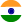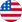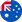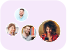24*7 support on WhatsAppChat NowProduct Edition:1st Edition
Author: Hossein Pishro-Nik
Book Name: Introduction To Probability, Statistics, And Random Processes
Subject Name: Maths

# Introduction To Probability, Statistics, And Random Processes 1st Edition Solutions

0 out of 5.0
57 reviews45 Students
have requested for homework help from this book

This book introduces students to probability, statistics, and stochastic processes. It can be used by both students and practitioners in engineering, various sciences, finance, and other related fields. It provides a clear and intuitive approach to these topics while maintaining mathematical accuracy. The book covers: Basic concepts such as random experiments, probability axioms, conditional probability, and counting methods Single and multiple random variables (discrete, continuous, and mixed), as well as moment-generating functions, characteristic functions, random vectors, and inequalities Limit theorems and convergence Introduction to Bayesian and classical statistics Random processes including processing of random signals, Poisson processes, discrete-time and continuous-time Markov chains, and Brownian motion Simulation using MATLAB and R (online chapters) The book contains a large number of solved exercises. The dependency between different sections of this book has been kept to a minimum in order to provide maximum flexibility to instructors and to make the book easy to read for students. Examples of applicationsâ€”such as engineering, finance, everyday life, etc.â€”are included to aid in motivating the subject. The digital version of the book, as well as additional materials such as videos

5
29
4
11
3
4
2
0
1
13

0

## Students who viewed this book also checked out